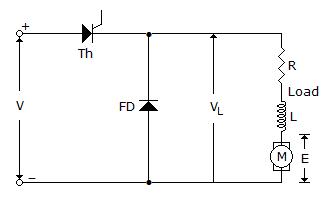# Test: Power Electronics - 4

## 25 Questions MCQ Test Power Electronics | Test: Power Electronics - 4

Description
Attempt Test: Power Electronics - 4 | 25 questions in 25 minutes | Mock test for Electrical Engineering (EE) preparation | Free important questions MCQ to study Power Electronics for Electrical Engineering (EE) Exam | Download free PDF with solutions
QUESTION: 1

Solution:
QUESTION: 2

Solution:
QUESTION: 3

### In a thyristor the gate lead is connected to the n layer near the p layer to which anode is connected.

Solution:
QUESTION: 4

A single phase half wave rectifier circuit has a free wheeling diode. The free wheeling diode will conduct only if

Solution:
QUESTION: 5

McMurray Bedford full bridge inverter uses

Solution:
QUESTION: 6

Which converter is used in HVDC transmission?

Solution:
QUESTION: 7

Assertion (A): The turn on time of SCR is about 1 to 4 ms.

Reason (R): The turn off time of SCR is about 10 to 300 ms.

Solution:
QUESTION: 8

An n pulse rectifier is fed by a source having an inductance L. If load current is I0, the voltage drop due to overlap is

Solution:
QUESTION: 9

Which chopper circuit operates in all the 4 quadrants?

Solution:
QUESTION: 10

A single phase cycloconverter feeds a resistance heating load. The heating power is provided by

Solution:
QUESTION: 11

A 3 phase fully controlled bridge converter is fed by a 3 phase system having phase voltage v = Vm sin ωt. The firing angle is a. The dc output voltage is

Solution:
QUESTION: 12

Which of these commutation methods uses an external pulse for commutation?

Solution:
QUESTION: 13

A separately excited dc motor is run from a dc source through a chopper. At 600 rpm and rated torque, the duty cycle of chopper is 0.8. At 600 rpm and half the rated torque, the duty cycle is likely to be

Solution:
QUESTION: 14

In a single phase full wave regulator feeding an R-L load, the power factor angle of load is θ. The range of control of firing angle a is

Solution:
QUESTION: 15

A relaxation oscillator uses

Solution:
QUESTION: 16

In a 3 phase bridge inverter with 180º mode of operation the numbers of thyristors conducting at one time are

Solution:
QUESTION: 17

A single phase full converter can operate in

Solution:
QUESTION: 18

In a 3 phase semiconverter, the freewheeling diode comes into operation only if firing angle

Solution:
QUESTION: 19

In the circuit of figure, the load current when thyristor is offSolution:
QUESTION: 20

A full bridge inverter is fed by a battery of 30 V. The rms value of output voltage is

Solution:
QUESTION: 21

In brushless excitation system of modern alternators, the main exciter is

Solution:
QUESTION: 22

The two-transistor model of a thyristor consists of two transistors :

Solution:
QUESTION: 23

In a thyristor, the forward breakover voltage

Solution:
QUESTION: 24

The gate lead, in a thyristor is welded to :

Solution:
QUESTION: 25

In a 3 phase full converter, the firing angle is less than 60º. The instantaneous output voltage

Solution:Use Code STAYHOME200 and get INR 200 additional OFF Use Coupon Code